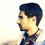# Statement Clarification for Dummies

This is a problem I often face. Sometimes I don't even understand what the problem statements say. It becomes frustrating when I see that many people have solved the problem already while I'm still struggling to understand what to prove or what to find.I associate this inability of mine with my unfamiliarity with many topics. However, I think I need to learn the art of understanding problems. Therefore, I seek guidance from fellow Brilliants. And, thus comes the real question!

Should we make a thread where people can post links to the problems they find ambiguous so that others can help?

# HelpNote by Atomsky Jahid
3 years, 6 months ago

This discussion board is a place to discuss our Daily Challenges and the math and science related to those challenges. Explanations are more than just a solution — they should explain the steps and thinking strategies that you used to obtain the solution. Comments should further the discussion of math and science.

When posting on Brilliant:

• Use the emojis to react to an explanation, whether you're congratulating a job well done , or just really confused .
• Ask specific questions about the challenge or the steps in somebody's explanation. Well-posed questions can add a lot to the discussion, but posting "I don't understand!" doesn't help anyone.
• Try to contribute something new to the discussion, whether it is an extension, generalization or other idea related to the challenge.

MarkdownAppears as
*italics* or _italics_ italics
**bold** or __bold__ bold
- bulleted- list
• bulleted
• list
1. numbered2. list
1. numbered
2. list
Note: you must add a full line of space before and after lists for them to show up correctly
paragraph 1paragraph 2

paragraph 1

paragraph 2

[example link](https://brilliant.org)example link
> This is a quote
This is a quote
    # I indented these lines
# 4 spaces, and now they show
# up as a code block.

print "hello world"
# I indented these lines
# 4 spaces, and now they show
# up as a code block.

print "hello world"
MathAppears as
Remember to wrap math in $$ ... $$ or $ ... $ to ensure proper formatting.
2 \times 3 $2 \times 3$
2^{34} $2^{34}$
a_{i-1} $a_{i-1}$
\frac{2}{3} $\frac{2}{3}$
\sqrt{2} $\sqrt{2}$
\sum_{i=1}^3 $\sum_{i=1}^3$
\sin \theta $\sin \theta$
\boxed{123} $\boxed{123}$

Sort by:

There is a report feature that you can request for more clarification.

- 3 years, 6 months ago

Sometimes I don't think reporting is an option. For example, take a look at this problem. I don't understand what this expression means. However, there are 29 solvers of the problem at this moment. So, I assumed the problem setter used some convention that I don't know of. The problem also doesn't have any link to a relevant wiki. I even tried to google this format to no avail. Is it really a good idea to report a problem which is not wrong (assumption, of course) in any way?

- 3 years, 6 months ago

"For 0<= y<= 1, find the maximum value of the absolute value of (y^2-xy) for constant x. Now, this maximum value should be a function with respect to x. Find the minimum value of this function for real x"

- 3 years, 6 months ago

Thanks! I have solved the problem after understanding it. :)

- 3 years, 6 months ago

The author seems to assume that readers are familiar with that expression. You can post a note like this to ask for clarification. Brilliant users will answer your query. In addition, if the moderators think that such clarification is necessary, they will add it to the problem statement, so other users can benefit from it as well.

For your problem, you want to minimise a function $f(x)$ for $x\in R$, and the function is $f(x) = \max_{0\leq y \leq 1} |y^2-xy|$. For example, when $x=0$, $f(x) = \max_{0\leq y \leq 1} |y^2-xy| = 1$.

- 3 years, 6 months ago

Thanks for your clarification! I have solved it afterward.

- 3 years, 6 months ago